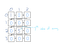# CS Data Structures: 2D ArrayPhoto by Sharon McCutcheon on Unsplash

# Two-Dimensional (2D) Array

`int bees;`
`bees;`

## 2D Array in Swift

`public struct Array2D<T> {    // 1.    public let columns: Int    public let rows: Int    private var array: [T]    // 2.     public var count: Int {        return array.count    }    // 3.     public init(columns: Int, rows: Int, initialValue: T) {        self.columns = columns        self.rows = rows        array = .init(repeating: initialValue, count: columns * rows)    }  }`
`array = .init(repeating: initialValue, count: columns * rows)`
`var bees = Array2D<Int>(columns: 3, rows: 4, initialValue: 0)`
`print(bees)// Array2D<Int>(columns: 3, rows: 4, array: [0, 0, 0, 0, 0, 0, 0, 0, 0, 0, 0, 0])`
`public subscript(column: Int, row: Int) -> T {    get {        precondition(column < columns, “Column Index (\(column)) is out of range. Array<T>(columns: \(columns), rows: \(rows))”)        precondition(row < rows, “Row Index (\(row)) is out of range. Array<T>(columns:\(columns), rows:\(rows))”)        return array[row*columns + column]    }    set {        precondition(column < columns, “Column Index (\(column)) is out of range. Array<T>(columns: \(columns), rows: \(rows))”)        precondition(row < rows, “Row Index (\(row)) is out of range. Array<T>(columns:\(columns), rows:\(rows))”)        array[row*columns + column] = newValue    }}`
`public struct Array2D<T> {    // 1.    public let columns: Int    public let rows: Int    private var array: [T]    // 2.    public var count: Int {        return array.count    }    // 3.    public init(columns: Int, rows: Int, initialValue: T) {        self.columns = columns        self.rows = rows        array = .init(repeating: initialValue, count: columns * rows)    }    public subscript(column: Int, row: Int) -> T {        get {            precondition(column < columns, “Column Index (\(column)) is out of range. Array<T>(columns: \(columns), rows: \(rows))”)            precondition(row < rows, “Row Index (\(row)) is out of range. Array<T>(columns:\(columns), rows:\(rows))”)            return array[row*columns + column]        }         set {            precondition(column < columns, “Column Index (\(column)) is out of range. Array<T>(columns: \(columns), rows: \(rows))”)            precondition(row < rows, “Row Index (\(row)) is out of range. Array<T>(columns:\(columns), rows:\(rows))”)            array[row*columns + column] = newValue        }    }}`

## Obtaining the Item at The Index Provided

`var bees = Array2D<Int>(columns: 3, rows: 4, initialValue: 0)print(bees)// Array2D<Int>(columns: 3, rows: 4, array: [0, 0, 0, 0, 0, 0, 0, 0, 0, 0, 0, 0])`
`bees[0, 0] = 1bees[1, 2] = 5print(bees)// Array2D<Int>(columns: 3, rows: 4, array: [1, 0, 0, 0, 0, 0, 0, 5, 0, 0, 0, 0])`Progression of the index in 2D Array
`let firstBee = bees[0, 0]print(firstBee)// 1let seventhBee = bees[1, 2]print(seventhBee)// 5`

## Remove Objects

`public mutating func removeLast() {    array.removeLast()}public mutating func removeAt(column: Int, row: Int) {    array.remove(at: row*columns + column)}`

## The Final Structure

`public struct Array2D<T> {    public let columns: Int    public let rows: Int    private var array: [T]    public var count: Int {        return array.count    }    public init(columns: Int, rows: Int, initialValue: T) {        self.columns = columns        self.rows = rows        array = .init(repeating: initialValue, count: columns * rows)    }    public subscript(column: Int, row: Int) -> T {        get {            precondition(column < columns, “Column Index (\(column)) is out of range. Array<T>(columns: \(columns), rows: \(rows))”)            precondition(row < rows, “Row Index (\(row)) is out of range. Array<T>(columns:\(columns), rows:\(rows))”)            return array[row*columns + column]        }        set {            precondition(column < columns, “Column Index (\(column)) is out of range. Array<T>(columns: \(columns), rows: \(rows))”)            precondition(row < rows, “Row Index (\(row)) is out of range. Array<T>(columns:\(columns), rows:\(rows))”)            array[row*columns + column] = newValue        }    }    public mutating func removeLast() {        array.removeLast()    }    public mutating func removeAt(column: Int, row: Int) {        array.remove(at: row*columns + column)    }}`